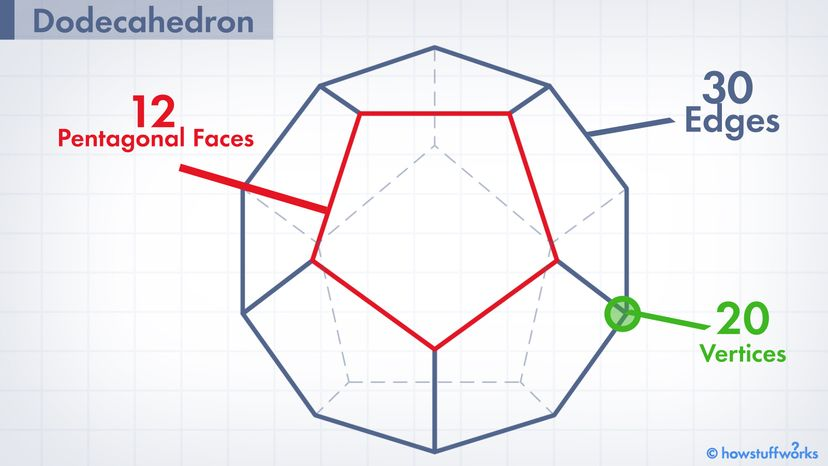# Dodecahedron: The 12-sided Shape With the 12-letter NameDodecahedrons are three-dimensional bodies containing a dozen flat faces — all shaped like pentagons. HowStuffWorks

Learning a new word every day is a pretty good habit to adopt. (Garfield's owner sure thinks so). And in that spirit, here's a fun math term you might not be aware of: "Dodecahedron." What's it mean? We're glad you asked. Here are 12 things to know about it.

Contents

## 1. Dodecahedrons Are a Kind of Polyhedron

Triangles and squares are both polygons, two-dimensional shapes formed with straight lines. Now just for kicks, let's go ahead and add a third dimension. A polyhedron is a 3-D object made up of polygonal faces. So while a square is a polygon, a cube is a polyhedron. Remember that for your next wine and cheese party ("Hey, can I get some more of those cheddar polyhedrons over here?").

## 2. Dodecahedrons Have 12 Pentagonal Faces

As you'll know if you've ever been to Washington, D.C., pentagons are 2-D shapes with five straight edges that form five angles at the corners. Dodecahedrons (pronounced dow·deh·kuh·hee·druhns) are three-dimensional bodies containing a dozen flat faces — all shaped like pentagons.

## 3. The Name "Dodecahedron" Has Greek Roots

Time to make the dad from "My Big Fat Greek Wedding" smile. "Dodecahedron" comes from the Greek words "dodeka" and "hedra," which mean "12" and "seat or base," respectively.

## 4. Each One Contains "Edges" and "Vertices"

"Edges" are a no-brainer; they're the lines where two of the polygons (squares, triangles, etc.) on a polyhedron meet. Vertices — or "vertexes" — are those pointy bits at which three or more faces converge. Every dodecahedron with identical faces will have 30 edges and 20 vertices.

## 5. The So-Called "Regular" Dodecahedron Is Part of an Elite Group ...

Check your scatology jokes at the door. A polyhedron is considered "regular" if (a) all its faces are identical polygons; (b) all the corners look the same; and (c) the edges are equal in length. Mathematicians have proven that there are only five kinds of regular polyhedrons. Together, they're known as the five "Platonic solids." And the regular dodecahedron happens to be one of them.

## 6. ... But It's Not Necessarily the Showiest Member

Cubes (aka: "regular hexahedrons") are the most famous Platonic solids, hands-down. You may also recognize the tetrahedron, a pyramid with a triangular base. Next up is the octahedron, an eight-faced beauty that sort of looks like a diamond. Finally, there's the icosahedron with its 20 — count 'em, 20 — triangular faces.

## 7. You Could Fill One Up With Other Platonic Solids

Here's a cool trick: If you merge five tetrahedrons together and use the layout of their vertices to create new polygonal faces, you just might get a perfect dodecahedron. By the same token, there are a few different ways to use compounds of cubes as the underpinnings for dodecahedrons. Spatial shenanigans are loads of fun.

## 8. Dodecahedrons Can Do Things Other Shapes Can't

If you lived on a giant dodecahedron, how would you navigate from Point A to Point B? Setting a course might be easier than you'd think. In 2018, mathematicians Jayadev Arthrea, David Aulicino and Pat Hooper showed it's possible to draw a straight pathway on a dodecahedron that begins at one vertex and returns to the same point — without turning or passing through any other corners. However, there'd be no way to accomplish this feat on a cube, tetrahedron, octahedron or icosahedron.

## 9. The Ancient Romans Used to Make Bronze Dodecahedrons

Since 1739, archeologists have unearthed dozens of small, man-made dodecahedrons at sites once occupied by the Roman Empire. Commonly made with bronze, these objects are hollow, they've got knobs on the vertices, and each face has a circular hole at its center. The specimens recovered thus far were built between the years 1 and 500 C.E. Opinions differ about their function; for all we know, the little oddities could've been weapons, dice, candle holders or something else altogether.

## 10. A Dodecahedron-shaped Life Form Existed 100 Million Years Ago

Braarudosphaera bigelowii was a species of algae that lived during the Age of Dinosaurs. Found in geologic sites all over the world, the microorganism was covered in pentagonal scales arranged together as a dodecahedron. Too bad we've never found a dinosaur with that kind of wild body plan.

## 11. "Dungeons and Dragons" Players Use Dodecahedron Dice

Participants roll die of many shapes and sizes in this role-playing fantasy game. The D12 dice, a go-to tool for many situations that involve big weapons, are mini dodecahedrons.

## 12. Salvador Dali's "The Sacrament of the Last Supper" Has a Dodecahedron in the Background

Since Christ had a dozen disciples, and there are a dozen months in the year, Dali felt "the 12 pentagons of a dodecahedron" would be a perfect fit (thematically) for this surreal painting he completed in 1955.